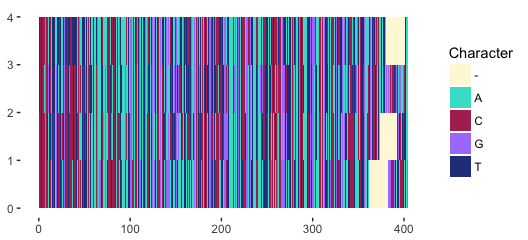Introduction to alignfigR

2018-07-03

The package alignfigR, built around ggplot2, creates multiple sequence alignment figures in R. In particular, alignfigR turns your sequence alignment into a ggplot object, which you can subsequently label, mark up, and save to your heart’s content.

General usage

As usual, load the library with either require or library. To input your alignment, use the function read_alignment(). Currently, only FASTA format is supported. The example file below contains DNA sequences, although any sequence alphabet (e.g. RNA and protein) is allowed.

require(alignfigR)
filename <- system.file("extdata", "example.fasta", package = "alignfigR")
my_data <- read_alignment(filename)

Then, simply create an alignment figure with the function plot_alignment(). This function takes two ordered arguments:

1. alignment, which corresponds to your sequence data set
2. palette, which gives the color-mapping scheme scheme for your sequence data. Several options exist for this argument, as follows.

Here are some examples for using the default color schemes in plot_alignment().

# Default DNA colors
plot_alignment(my_data, "dna")

# Default RNA colors
plot_alignment(my_data, "dna")

# Default protein colors
plot_alignment(my_data, "protein")

# Random colors are the default. Either provide the argument "random", or simply provide nothing.
plot_alignment(my_data) # Or, this code:  plot_alignment(my_data, "random")

You can also specify your own color scheme using a named-array, as follows. Note that missing characters (such as gaps) can also be colored. This option is particularly useful for dealing with noncanonical data (e.g. binary or character data). However, word of caution! If any alignment chartacters are not assigned a color, such characters will be left as whitespace in the resulting plot.

my_favorite_colors <- c("A" = "pink", "C" = "magenta", "G" = "seagreen", "T" = "yellow",
"-" = "black")
p <- plot_alignment(my_data, my_favorite_colors)

Finally, let’s use the default color scheme to plot this DNA alignment.

p <- plot_alignment(my_data, "dna")
p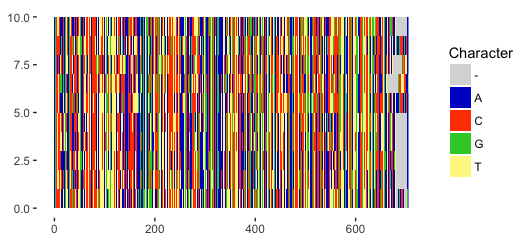As mentioned, you can manipulate this figure in any way you want, using ggplot2, from here on out. For instance, maybe a title!

p + ggtitle("My fancy-schmancy alignment figure!")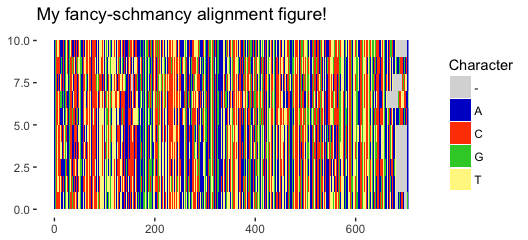Plotting alignment subsets

By default, plot_alignment() will create a figure for your entire alignment. However, it is also possible to plot only a subset of your alignment, selecting particular taxa and/or columns.

To restrict the plot to certain taxa, specify the taxa you’d like to keep with the argument “taxa”.

plot_alignment(my_data, "dna", taxa = c("Cow", "Carp"))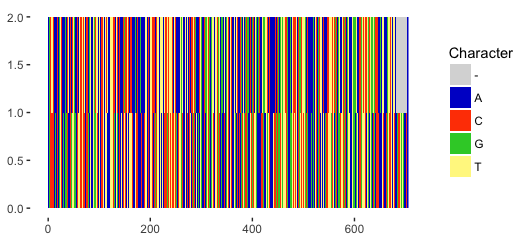You can alternatively exclude specified taxa from the plot by adding the argument exclude_taxa = T.

plot_alignment(my_data, "dna", taxa = c("Cow", "Carp"), exclude_taxa = T)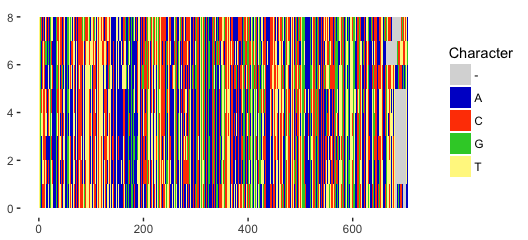Columns can be similarly specified with the argument “columns”.

plot_alignment(my_data, "dna", columns = c(1:25))And again, you can instead exclude specific columns by adding the argument exclude_columns = T.

plot_alignment(my_data, "dna", columns = c(1:200), exclude_columns = T)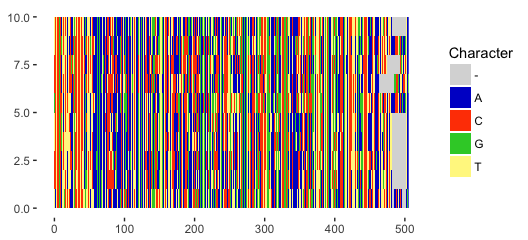And of course, we can also combine these options do get any alignment subset we want (with exciting colors, too)!

exciting_colors <- c("A" = "turquoise", "C" = "maroon", "G" = "mediumpurple1", "T" = "royalblue4", "-" = "cornsilk1")
plot_alignment(my_data, exciting_colors, columns = c(1:200, 350:450), exclude_columns = T, taxa = c("Cow", "Carp", "Chicken", "Human") )